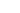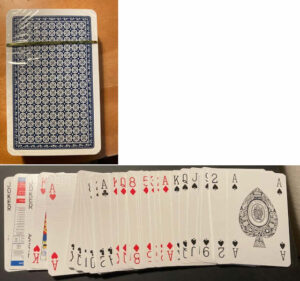Tag: deckMore on Shuffledness

So I’ve updated my shuffledness calculation. This is a bit of a work in progress so probably isn’t the last version.  One of the things I’m interested in is what is the optimal number of swaps to get a good shuffled deck of cards. The shuffle algorithm picks two random indexes in the array 0-51 and then swaps the contents.

Obviously doing this 10 times would only shuffle at most 20 cards and probably fewer as there’s no checks for repeats. The only check is that both indexes (indices?) are not the same. So I want to experiment and see what an optimal value for numTries should be..

void ShuffleDeck(int numtries) {
int firstIndex, secondIndex;
for (int i = 0; i < numtries; i++) {
do {
firstIndex = rand() % 52;
secondIndex = rand() % 52;
}
while (firstIndex == secondIndex);
int value = deck[firstIndex];
deck[firstIndex] = deck[secondIndex];
deck[secondIndex] = value;
}
}

Anyway, I’ve added a CalcDistance() function. This looks at each card and calculates how far it has moved from it’s original position.  It then divides this by the maximum distance it could have moved to get a move “factor”. These are summed up and divided by 52 to give an average move factor. That’s your CalcDistance() function.

float CalcDistance() {
float distance = 0.0f;
for (int i = 0; i < 52; i++) {
float distanceDiv = (i < 26) ? 51 - i : i-1;
//printf("%i: %3.0f\n", i, distanceDiv);
distance += (abs(deck[i] - i) / distanceDiv);
}
return distance/52.0f;
}

The only thing odd about this function is the distanceDiv value which starts at 51, decreases to 25 then increases to 50. The printf let me check that was correct.

This is the current state of the program which I’ve listed fully below. Enjoy! It’s 63 lines long.

// shuffledness.c : This measures how shuffled a deck of cards is
// cards are hjeld as values 0-51 in a 52 int aaray.

#include <time.h>
#include <stdio.h>
#include <stdlib.h>

int deck;
time_t t;

// Order the cards 0-51. 0 = Acew Hards,13 = Ace Clubs,26 = Ace of Diamonds and 51 = King of Spades
void InitDeck() {
for (int i = 0; i < 52; i++) {
deck[i] = i;
}
}

// Works on glovbal array deck and calculates a value for how displayed each card is
float CalcDisorder() {
int total=0;
for (int i = 1; i < 52; i++) {
total += abs(deck[i] - deck[i - 1]);
}
printf("Total = %d\n", total);
}

// Sum up distance card moved/max distance
float CalcDistance() {
float distance = 0.0f;
for (int i = 0; i < 52; i++) {
float distanceDiv = (i < 26) ? 51 - i : i-1;
//printf("%i: %3.0f\n", i, distanceDiv);
distance += (abs(deck[i] - i) / distanceDiv);
}
return distance/52.0f;
}

// Shuffle a deck by swapping two random cards a specified number of times
void ShuffleDeck(int numtries) {
int firstIndex, secondIndex;
for (int i = 0; i < numtries; i++) {
do {
firstIndex = rand() % 52;
secondIndex = rand() % 52;
}
while (firstIndex == secondIndex);
int value = deck[firstIndex];
deck[firstIndex] = deck[secondIndex];
deck[secondIndex] = value;
}
}

int main() {
/* Intializes random number generator */
srand((unsigned)time(&t));
for (int i = 0; i < 25; i++) {
InitDeck();
ShuffleDeck(1000);
printf("CalcDisorder() ==%f x %f = %f\n", CalcDisorder(),CalcDistance(),(double)CalcDisorder() * CalcDistance());
}
return 0;
}

Because I’m multiplying the two factors (disorder and Distance, the final value is never getting much above 0.35 and I’d prefer it to be in the range 0-0.999. Suggestions welcomed.

.How to measure how shuffled a deck of cards isI first thought about this when I wrote the program to shuffle  deck of cards using a riffle shuffle. If you are given a deck of cards (or pack of cards as us Brits say), how do you discern just how shuffled the pack is? Can you calculate a numeric value for it say a % ranging from 0 to 100?

I believe it’s possible.  Here’s how.

1. Start with a default pack of cards in perfect sorted order. Out of curiosity I found an unopened deck oif Waddington’s cards and opened it as the photos show. The cards in the pack were arranged in order King, Queen, Jack down to Ace in each of the four suits Heart, Clubs, Diamonds and Spades in that order. Let’s reverse the card rank ordering so a full deck starts with Ace Hearts through to King Hearts, Ace of Clubs to King of Clubs and so on with the last card being the King of Spades.
2. Instead of referring to cards by their rank and suite lets just number them 0-51. 0= Ace of Hearts, 51 = King of Spades.
3. When a deck is shuffled, each card can move to any other position. So a measure of shuffledness is calculating how far the cards moved in aggregate.
4. However the card movements have to be “normalized”. Cards 0 and 51 can move to any of 51 positions while cards 26 (King of Clubs) and 27 (Ace of Diamonds) can only move a maximum of 26 places.
5. I’m looking at the absolute value of a movement so if card 3 moves to position 47, it has moved 44 places and likewise card 47 moving to position 3 moves 44 (not -44) places.
6. So to normalize a card’s move, divide its move by its maximum possible distance it can move. So wherever card 0 moves divide it by 51, card 1 by 50, card 26 ‘ move by 26.
7. Sum up all 52 normalized move’s and multiply by 100. That is the measure of shuffledness.

I’ll write a C program to measure how shuffled a deck is and publish it in a day or two. Also here is a conversation on Reddit about shuffling cards.